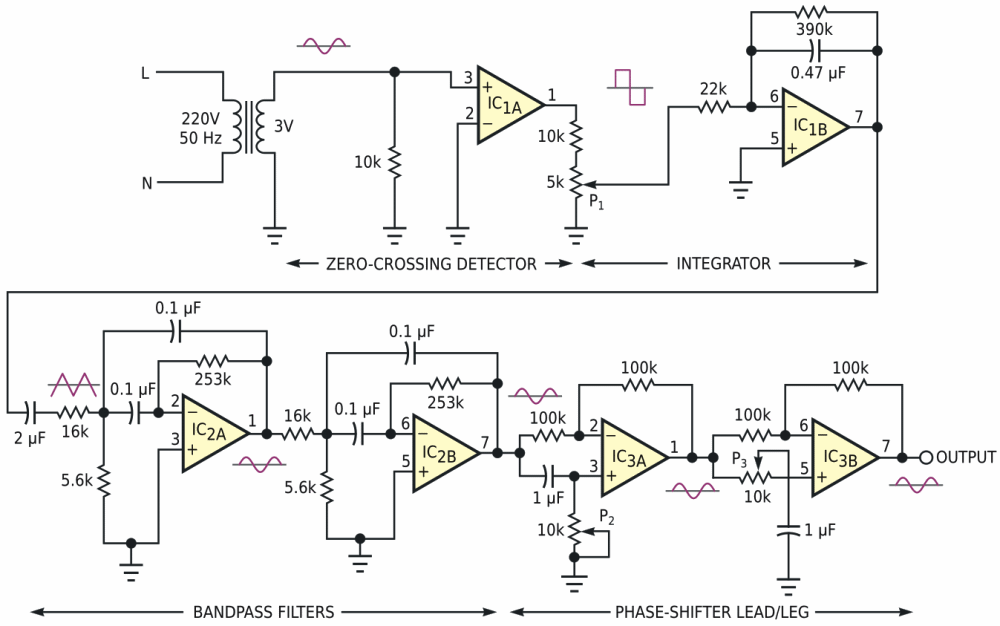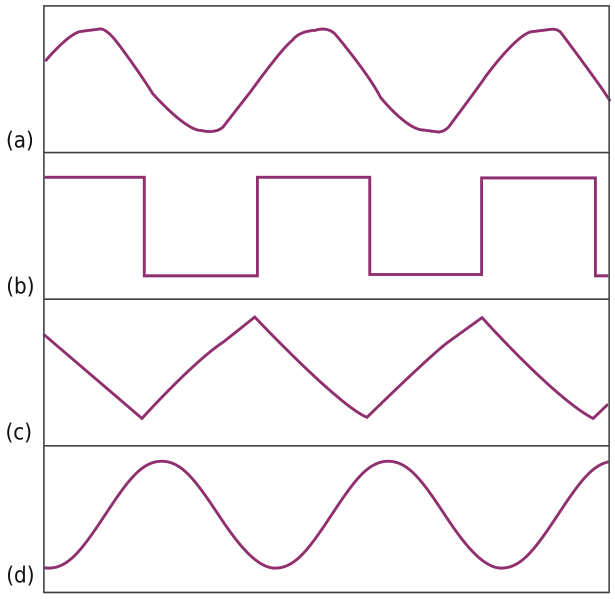# Sine reference is synchronous with ac line

## Texas Instruments LM358

Many applications require a sinusoidal reference voltage synchronized to the ac line voltage. You cannot derive such a reference voltage directly from the ac line because the waveform of the ac line is distorted because of nonlinear loads connected to the line and because the amplitude of the line signal varies. Hence, you cannot use a simple step-down transformer to derive the reference signal.

The circuit in Figure 1 develops a line-synchronized reference signal whose phase and amplitude you can adjust using potentiometers P1, P2, and P3. The circuit steps down and converts the line voltage to a square wave using the IC1A zero-crossing detector. The integrator block, IC1B, then integrates the square wave to produce a triangular wave. The triangular wave passes through two narrow bandpass filters comprising IC2A and IC2B. The center frequency of the bandpass filters matches the 50-Hz line frequency. The sine-wave output from the filters passes through two phase shifters to set the phase at either lead or lag. The allpass-filter configuration comprising IC3A and IC3B sets the phase of the reference signal. You can adjust the amplitude of the output using potentiometer P1, and you can adjust the phase with P2, P3, or both. The circuit uses three low-power LM358 dual op amps.Figure 1. An op-amp circuit uses only resistors and capacitors to generate a line-synchronized sine wave.

Figure 2 shows the measured waveforms at different points in the circuit. The reference sine wave has total harmonic distortion of 0.7%. Note that the circuit requires no heavy, bulky inductors.Figure 2. Distorted line voltage (a) produces a square wave (b). An integrator creates a triangular wave (c), and bandpass filters produce a pure sine wave (d).

EDN